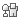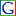# Multilingual dictionary of abbreviations

• Suggest an abbreviation
• Search an abbreviation
•# R

Code

Meaning

Language

Themes and places

R
Arginine

= R | Arginina | In Catalan
= R | Arginin | In Czech
= R | Arginin | In German
= R | Arginina | In Spanish
= R | Arginino | In Esperanto
= R | Arginine | In French
= R | 아르기닌 | In Korean
= R | Arginin | In Croatian
= R | Arginin | In Indonesian
= R | Arginina | In Italian
= R | ארגינין | In Hebrew
= R | Arginīns | In Latvian
= R | Arginin | In Letzeburgesh
= R | Argininas | In Lithuanian
= R | Arginine | In Dutch
= R | アルギニン | In Japanese
= R | آرګېنين | In Pashto
= R | Arginina | In Polish
= R | Arginina | In Portuguese
= R | Аргинин | In Russian
= R | Arginín | In Slovak
= R | Arginiini | In Finnish
= R | Arginin | In Swedish
= R | Arginin | In Turkish
= R | Аргінін | In Ukrainian
= R | 精氨酸 | In Chinese languages

In English

R
Arginina

= R (look above)

In Catalan

R
Arginin

= R (look above)

In Czech

R
Arginin

= R (look above)

In German

R
Arginina

= R (look above)

In Spanish

R
Arginino

= R (look above)

In Esperanto

R
Arginine

= R (look above)

In French

R
아르기닌

= R (look above)

In Korean

R
Arginin

= R (look above)

In Croatian

R
Arginin

= R (look above)

In Indonesian

R
Arginina

= R (look above)

In Italian

R
ארגינין

= R (look above)

In Hebrew

R
Arginīns

= R (look above)

In Latvian

R
Arginin

= R (look above)

In Letzeburgesh

R
Argininas

= R (look above)

In Lithuanian

R
Arginine

= R (look above)

In Dutch

R
アルギニン

= R (look above)

In Japanese

R
آرګېنين

= R (look above)

In Pashto

R
Arginina

= R (look above)

In Polish

R
Arginina

= R (look above)

In Portuguese

R
Аргинин

= R (look above)

In Russian

R
Arginín

= R (look above)

In Slovak

R
Arginiini

= R (look above)

In Finnish

R
Arginin

= R (look above)

In Swedish

R
Arginin

= R (look above)

In Turkish

R
Аргінін

= R (look above)

In Ukrainian

R
精氨酸

= R (look above)

In Chinese languages

R

Röntgen

In many languages